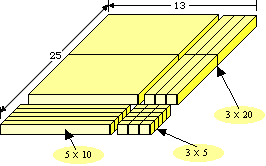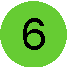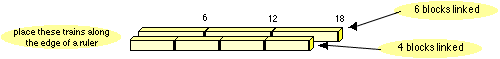# Base Ten Blocks

Objective: Demonstrate and describe the process of multiplication (3-digit by 1-digit), using manipulatives, diagrams and symbols.
Materials: Base ten blocks, paper, pencil, 5 x 8 cards.Use base ten blocks to construct a model of multiplication equations: To show 132 x 5 we would do the following: Take five of the 5" x 8" cards and spread them on the table top. On top of each of these cards place one flat block (100), 3 long blocks (30), and 2 single blocks (2) to create 132 (100 + 30 + 2). On a piece of paper write the equation 132 x 5, and begin the multiplication process. Remove the singles from each card (5 x 2), and trade up if possible (to 1 long). Remove the long blocks from each card (5 x 3), and trade up if possible (1 flat). Remove the flat blocks from each card, and trade up if possible. Read the product. Try these:   143 x 2   122 x 3   248 x 2   159 x 6

Objective: Estimate, mentally calculate, compute or verify, the product (3-digit by 2-digit) and quotient (3-digit divided by 1-digit) of whole numbers.
Materials: Base ten blocks, paper, pencil.

 Use the base ten blocks to model a 2-digit by 2-digit multiplication equation. To show 25 x 13, we would create a model like the one shown. When we multiply 25 by 13, we essentially repeat the multiplication process four times: 3 x 5, 3 x 20, 10 x 5, and 10 x 20. Can you find the products of each of these portions of the multiplication in the model given? Some for you to try: 23 x 14 18 x 12 24 x 35 16 x 14Objective: Recognize, model, identify, find and describe common multiples, common factors, least common multiple, greatest common factor and prime factorization, using numbers 1 to 100.
Materials: base ten blocks, cm ruler or metre stick.Assume you are trying to find the LCM of 4 and 6. Start by creating several "trains" of blocks 4 singles long and several which are 6 singles in length. Place these trains along the edge of the cm ruler starting at 0. Every place where one of the trains ends represents a multiple of that number. You will find that the multiples of 4 are 4, 8, 12, 16, 20, ... and the multiples of 6 are 6, 12, 18, 24, 30 ... Wherever the trains end at the same spot represents a "common" multiple. The first such instance is the "least" common multiple. A diagram is shown below. The least common multiple of 4 and 6 is 12. How could you model the "greatest common factor"?